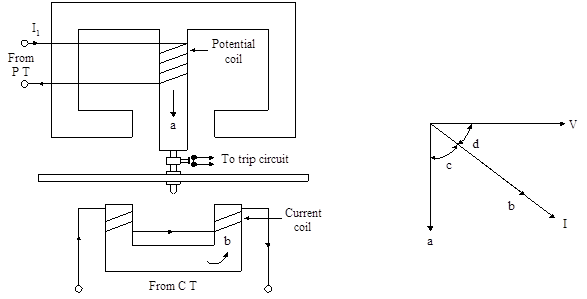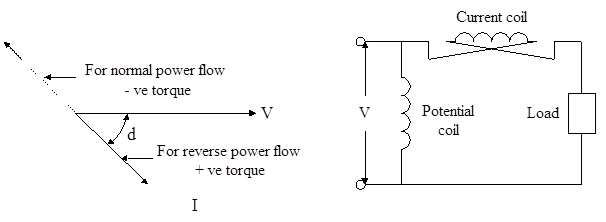### Induction Type Directional Power Relay or Reverse Power Relay

• This type of relay operates when power in the circuit flows in a specific direction. Unlike a non directional over-current relay (where the driving torque is due to the interaction of magnetic fields derived form current in the relay winding), a directional power relay is so designed that it obtains its operating torque by the interaction of magnetic fields derived from both voltage and current source of the circuit it protects. Thus this type of relay is essentially a wattmeter and the direction of the torque set up in the relay depends upon the direction of the current relative to the voltage with which it is associated.

Construction:

• Figure 2.15 (i) the essential parts of a typical induction type directional power relay. It consists of an aluminium disc which is free to rotate in between the poles of two electromagnets. The upper electromagnet carries a winding called potential coil on the central limb which is connected through a Potential Transformer (P.T.) to the circuit voltage source. The lower electromagnet has a separate winding called current coil connected to the secondary of Current Transformer (C.T.) in the line to be protected. The current coil is provided with a number of tappings connected to the plug setting bridge (not shown), which permits any desired current setting. The restraining torque is provided by a spiral spring.(i)                                                                                                     (ii)

a = Φ1= Flux 1; b = Φ2 = Flux 2; c = α; d = θ

Fig. 2.15 Directional power relay

• The spindle of the disc carries a moving contact which bridges two fixed contacts when the disc has rotated through a pre-set angle. By adjusting this angle, the travel of the moving disc can be adjusted and hence any desired time setting can be given to the relay.

Operation:

• The flux Φ1 due to current in the potential coil will be nearly 90o lagging behind the applied voltage V. The flux Φ2 due to the current coil will be nearly in phase with the operating current I [refer vector diagram 2.15 (ii)]. The interaction of fluxes Φ1 and Φ2 with the eddy currents induced in the disc produces a driving torque given by

T  α  Φ1  x  Φ2  x  sin α

Since,  Φ1    α    V,

Φ2   α   I   and

α  =  90 – θ

T α V I sin (90 – θ)

α V I cos θ

α power in the circuit.

• Torque produced is positive when cos θ is positive, i.e. θ is less than 90o. When θ is more than 90o (between 90o and 180o), the torque is negative.

• At a particular relay location, when power flows in the normal direction, the relay is connected to produce negative torque i.e. the driving torque and restraining torque (due to spring) help each other to turn away the moving contact from the fixed contacts. The angle between the actuating quantities supplied to the relays is kept (180o – θ) to produce negative torque. Consequently, the relay remains inoperative. If due to any reason like reverse power of Turbo Alternator (reverse power called non fault condition), the power flows in the reverse direction, the relay produces a positive torque and it operates. In this condition the angle between the actuating quantities θ is kept less than 90o to produce positive torque. This is shown in figure 2.16 (i). For normal flow of power, the relay is supplied with ‘V’ and ‘– I’. For reverse flow, the actuating quantities become ‘V’ and ‘I’. Torque becomes positive. This can be achieved easily by reversing the current coil, as shown in figure 2.16 (ii).(i)                                                                                                             (ii)

d = Φ

2.16 (i) Phasor diagram for power relay & (ii) Connection of current coil for power relay

• Relaying units supplied with single actuating quantities discussed earlier are non-directional over-current relays. Non directional relays are simple and less expensive than directional relays.